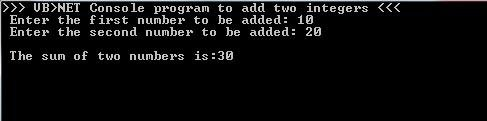# VB.NET PROGRAM TO ADD TWO NUMBERS / INTEGERS

In this post, we will see a VB.Net Program to read two number inputs from user and display the sum.

The program below accepts two numbers from user and perform basic arithmetic operation(addition) using `+` operator and display the sum on screen.

For algorithm & flowchart to add two numbers, go through the post given below.

### Program :

Sample Output :